Courses

# Long Answer Type Questions- Triangles Class 10 Notes | EduRev

## Class 10 : Long Answer Type Questions- Triangles Class 10 Notes | EduRev

The document Long Answer Type Questions- Triangles Class 10 Notes | EduRev is a part of the Class 10 Course Class 10 Mathematics by VP Classes.
All you need of Class 10 at this link: Class 10

Q1.Prove that the ratio of the areas of two similar triangles is equal to the ratio of squares of their corresponding sides.
Using the above result, prove the following:
In Δ ABC, XY is parallel to BC and it divides Δ ABC into two parts of equal area. Prove that:
=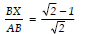Sol. Part-I  [See proof of the theorem on ratio of areas of similar triangles]
Part-II
We have a Δ ABC in which XY y BC such that
ar (Δ ABC) = 2 ar (Δ AXY)
⇒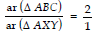...(1)
Now, in Δ ABC and Δ AXY, we have
<1 = <2  and  <3 = <4 [Corresponding angles]
⇒ Δ ABC ~ Δ AXY
[using the Basic Proportionality Theorem]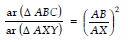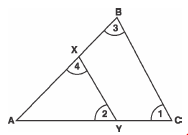[using the above theorem] ...(2)
From (1) and (2), we get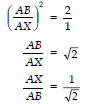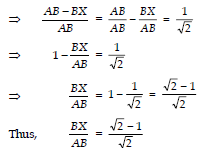Q2.Prove that the ratio of the areas of two similar triangles is equal to the ratio of the squares on their corresponding sides.Using the above, do the following:
The diagonals of a trapezium ABCD, with AB y DC, intersect each other at the point O. If  AB = 2 CD, find the ratio of the area of Δ AOB to the area of ΔCOD.
Sol.
Part-I  [See proof of the theorem on the ratio of areas of similar Ds
Part-II
We have a trapezium ABCD such that AB y DC and AB = 2 CD
In Δ AOB and Δ COD
Œ AB y DC
∴ <1 = < 2
[Int. alternate angles are equal]
<3 = <4
∴ Using AA similarity, we have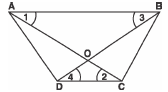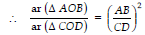But AB = 2 CD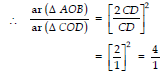Thus, ar (Δ AOB) : ar (Δ COD) = 4 : 1.

Q3. A peacock is sitting on the top of a pillar, which is 9 m high. From a point 27 m away from the bottom of the pillar, a snake is coming to its hole at the base of the pillar. Seeing the snake, the peacock pounces on it. If their speeds are equal, at what distance from the hole is the snake caught?
Sol.
Let the position of hole is at ‘H’ and snake be at ‘S’. If the point ‘P’ represents the peacock and let the distance between hole to the place where they meet = x metres
∴  SH = 27 m = PC
[Œ their speeds are equal]
Now SC = (27 - x) m
PC = (27 - x) m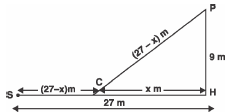In right D PHC, we have:
PH2 + CH2 = PC2
[By Pythagoras Theorem]
⇒ (9)2 + (x)2 = (27 - x)2
⇒ 81 + x2 = 729 - 54x + x2
⇒ 54x = 729 - 81 = 648
⇒ x = 648/54=12m
Hence, the required distance = 12 m.

Q4. Prove that the ratio of the areas of two similar triangles is equal to the ratio of squares of their corresponding sides.
Using the above result, prove the following:
If D is a point on the side AB of D ABC such that
AD : DB = 3 : 2 and E is a point on BC such that DE y AC; find the ratio of the areas of Δ ABC and Δ BDE.
Sol.
Part-I  [See proof of the theorem on ratio of areas of similar triangles]
Part-II
In Δ ABC,
Œ DE y AC [Given]
∴ <1 = <2 [Corresponding Angles]
Let BD = 2 k  and  AD = 3 k
∴ AB = AD + DB
= 3 k + 2 k = 5 k
Now in D ABC and D BDE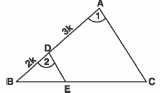<B = <B [Common]
<1 = <2 [Proved above]
∴ Using AA similarity we have:
Δ ABC ~ Δ BDE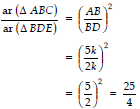⇒ ar (Δ ABC) : (ar Δ BDE) = 25 : 4.

Q5. Prove that in a right triangle the square on the hypotenuse is equal to the sum of the squares of the other two sides.
Making use of the above, prove the following:
In a rhombus ABCD, prove that:
4 AB2 = AC2 + BD2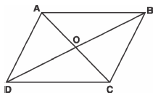Sol. Part-I . [see proof of Pythagoras Theorem]
Part-II.
We have a rhombus ABCD whose diagonals AC and BD intersect at O.
Since, the diagonals of a rhombus bisect each other at right angles.
∴ AC ≌ BD  And
OA = OC
OB = OD
In right Δ AOB, we have
AB2 = OA2 + OB2
[using the above result]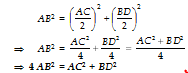Q6. Prove that the ratio of the areas of two similar triangles is equal to the square of the ratio of their corresponding sides.
Using the above, do the following:
If D is a point on the side AC of D ABC such that AD : DC = 2 : 3 and E is a point on BC such that DE y AB; then find the ratio of areas of Δ ABC and Δ CDE.
Sol.
Part-I  [See the proof of the theorem]
Part-II. We have D ABC in which
DE y AB
∴ In Δ ABC and Δ DEC,
<C = <C [Common]
<1 = <2 [Corresponding
Using AA similarity,
Δ ABC ~ Δ DEC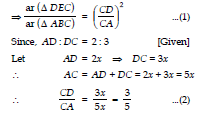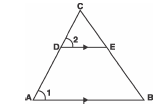From (1) and (2), we get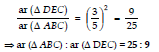Q7.Prove that, if a line is drawn parallel to one side of a triangle to intersect the other two sides in distinct points, the other two sides are divided in the same ratio.
Using the above result, do the following:
In the figure, DE y BC and BD = CE. Prove that D ABC is an isosceles triangle.
Sol.
Part-I  [See the proof of Basic Proportionality Theorem]
Part-II In Δ ABC,
Since, DE y BC [Given]
∴ Using the Basic Proportionality Theorem, we get
=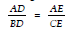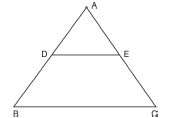From (1) and (2) we get
=From (2) and (3), we get
AD + BD = AE + CE
⇒ AB = AC
⇒ Δ ABC is an isosceles triangle.

Q8. Prove that, in a right triangle, the square of the hypotenuse is equal to the sum of the squares of the other two sides.
Using the above, do the following:
Prove that, in a D ABC, if AD is perpendicular to BC, then
AB2 + CD2 =   AC2 + BD2
Sol.
Part-I  See the proof of the Pythagoras Theorem]
Part-II  We have Δ ABC in which AD ⊥ BC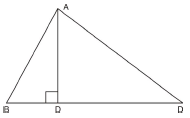∴ In right D ADB, <D = 90°
∴ Using Pythagoras theorem,
⇒AD2 = AB2 - BD2  ...(1)
⇒ AD2 = AC2 - CD2  ...(2)
From (1) and (2), we get
AB2 - BD2 = AC2 - CD2
AB2 + CD2 = AC2 + BD2

Q9. ABCD is a trapezium with AB y DC. E and F are points on non-parallel sides AD and BC respectively such that EF is parallel to AB. Show that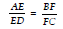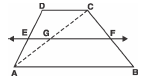Sol. Join AC such that it meets EF at G.
ABy DC and  EFy AB
∴ EF y DC [Q Lines parallel to the same line are parallel to each other]
EG y DC [Q EG is a part of EF]
⇒  =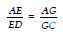...(1)
Next in DCAB
=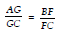...(2)
From (1) and (2), we get
=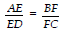Q10. ABCD is a trapezium in which AByDC and P and Q are points on AD and BC, respectively such that PQy DC. If PD = 18 cm, BQ = 35 cm and QC = 15 cm, find AD.
[NCERT Exemplar]
Sol.
Since AByDC and PQyDC
⇒  =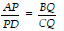...(1)
Substituting
PD = 18 cm,   BQ = 35 cm
and  CQ =  15 cm in (1), we get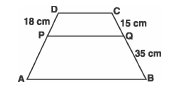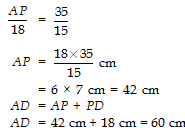Since, AD = AP + PD
∴ AD = 42 cm + 18 cm = 60 cm

Q11. In the following figure if AByDC and AC and PQ intersect each other at the point O, prove that:
OA×CQ = OC×AP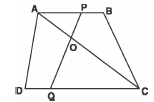Sol.
Q AB||DC and AC is a transversal,
∴  <OCQ = <OAB
[Alternate angles]
Again, PQ is also a transversal
∴ <OQC = <APO
[Alternate angles]
Now, in Δ  OAP and Δ OQC, we have
<OAP = <OCQ
<APO = <OQC [Proved above]
<AOP = <QOC [Vertically opposite angles]
⇒ Δ OAP and Δ  OQC are equiangular
∴ The triangles are similar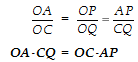Q12. Hypotenuse of a right triangle is 25 cm and out of the remaining sides, one is longer than the other by 5 cm. Find the lengths of the other two sides.
Sol.
Hypotenuse of the rt triangle = 25 cm
Let one of the sides be x cm
∴ Other side = (x + 5)cm
Now, using Pythagoras Theorem,
x2 + (x + 5)2 = 252
⇒ x2 + x2 + 10x + 25 = 625
⇒ 2x2 + 10x = 625 – 25 = 600
or x2 + 5x – 300 = 0
or x2 + 20x – 15x – 300 = 0
or x(x + 20) – 15 (x + 20)
or (x – 15) (x + 20) = 0
Either x – 15 = 0
⇒ x = 15
or x + 20 = 0
⇒ x = – 20
x = – 20
is not required.
Therefore, x = 15
Now, x + 5 = 15 + 5 = 20
The other two sides are 15 cm and 20 cm.

Q13. Prove that the ratio of the areas of two similar triangles is equal to the square of the ratio of their corresponding sides.
Using the above, do the following:
In a trapezium ABCD, AC and BD are intersecting at O, AB y DC and AB = 2 CD. If the area of Δ AOB = 84 cm2, find the area of Δ COD.
Sol.
Part-I  [See the proof of the theorem on areas of similar triangles]
Part-II  In Δ AOB and Δ COD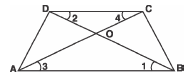<1 = <2
[Alternate interior angles Œ AB y DC]
<3 = <4
⇒ Δ AOB ~ Δ COD
[By AA similarity]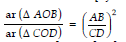[using the above theorem]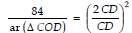[Œ It is given that AB = 2 CD]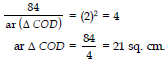Q14. In a triangle, if the square on one side is equal to the sum of the squares on the other two sides, prove that the angle opposite to the first side is a right angle. Use the above theorem to find the measure of <PKR in the figure.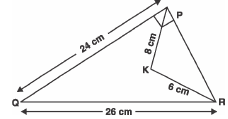Sol.
Part-I  [See the converse of the Pythagoras theorem]
Part-II  In right Δ QPR
Using Pythagoras theorem,
PR2 + PQ2 = QR2
⇒ PR2 + (24)2 = 262
⇒ PR2 = 262 - 242
= (26 - 24) (26 + 24)
= 2 × 50 = 100 ...(1)
Now in D PKR,
PK2 + KR2 = 82 + 62
= 64 + 36 = 100 ...(2)
From (1) and (2),
PK2 + KR2 = PR2
which is according to the above theorem.
∴Angle opposite PR must be 90°
⇒ <PKR = 90°

Offer running on EduRev: Apply code STAYHOME200 to get INR 200 off on our premium plan EduRev Infinity!

132 docs

,

,

,

,

,

,

,

,

,

,

,

,

,

,

,

,

,

,

,

,

,

;